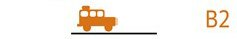Link to instructions and article: Click Here
Maximums
From Compliance Plates, Handbook or Manuals etc
Car
Weight
Max Front Axle Load (Car)
Max Rear Axle Load (Car)
Gross Vehicle Mass (GVM)
Gross Combination Mass (GCM)
Max Ball Weight (Car)
Trailer
Allowable Trailer Mass (ATM)
Gross Trailer Mass (GTM)
Actuals
Progressive weights of Car and Trailer over the weighbridge
Car and Trailer Hithced
Under / -Over
Progressive Weights Over the Weighbridge
Front Axle (Car) (Measured)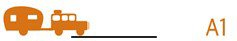Rear Axle (Car) (Calculated)
Whole Car (GVM) (Measured)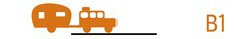Whole Car and Trailer (GCM) (Measured)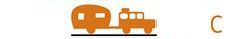Ball Weight (Calculated)
Trailer Mass (Calculated)
Ball Weight as % of Trailer Mass (Calculated)
Trailer Axle (GTM) (Measured)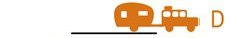Car Only
Progressive weights of Car only over the weighbridge
Front Axle (Car) (Measured)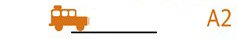Rear Axle (Car) (Calculated)
Whole Car (GVM) (Measured)